Like   Tweet

 table div table+table+table+table div table{width:100%;padding:0}table div table+table+table+table div table img{width:96.23%;padding:0;float:none}table div table+table+table+table div table td{width:100%;padding:0 1.88% 18px}/* styles */## Best of Black Harvest in the Chicago Public Library Series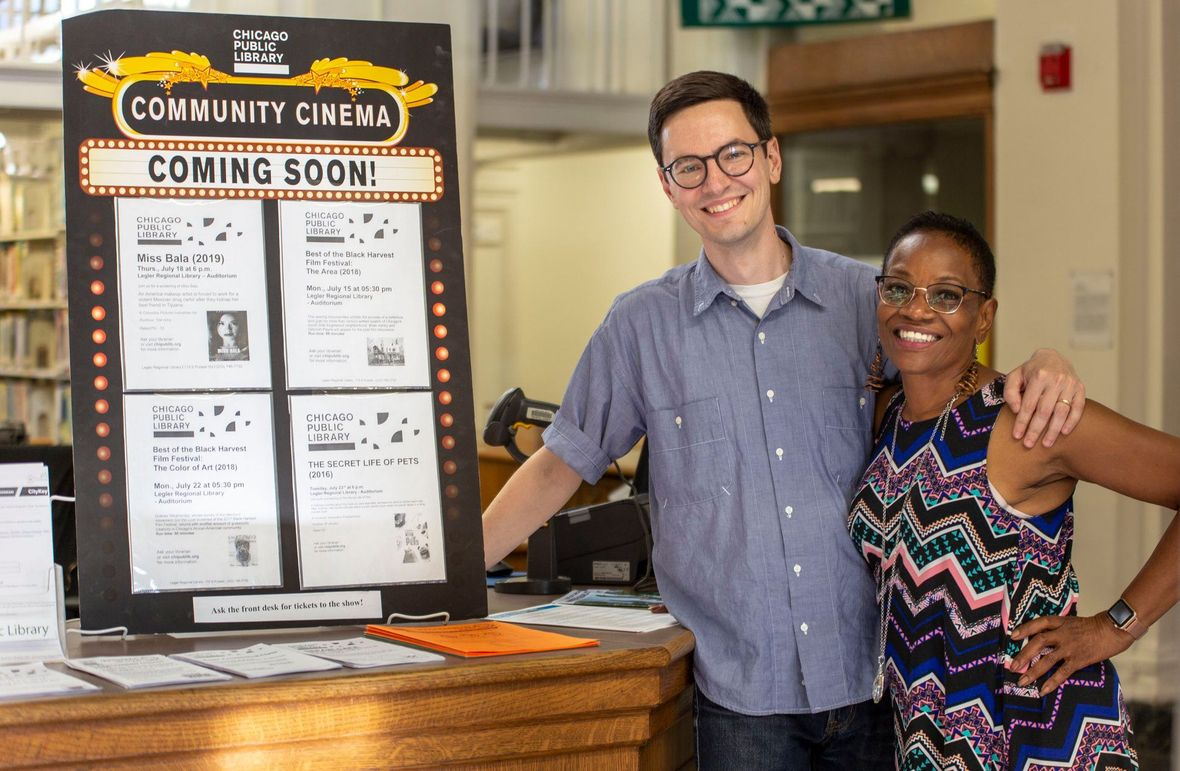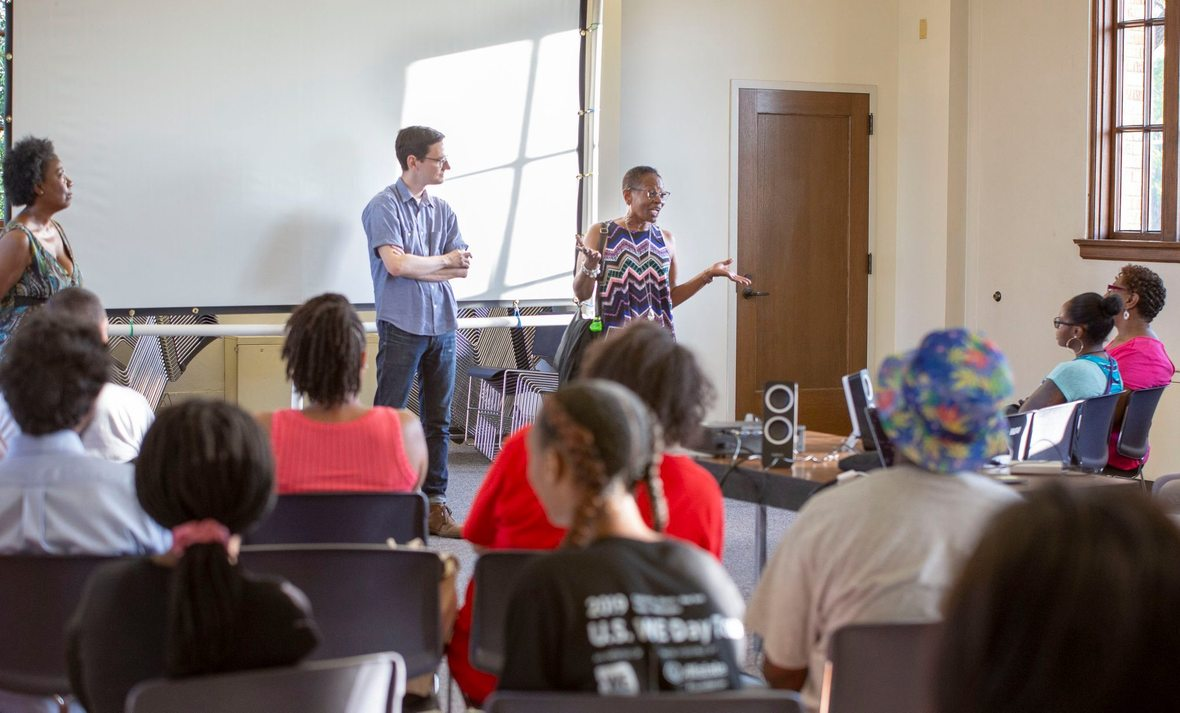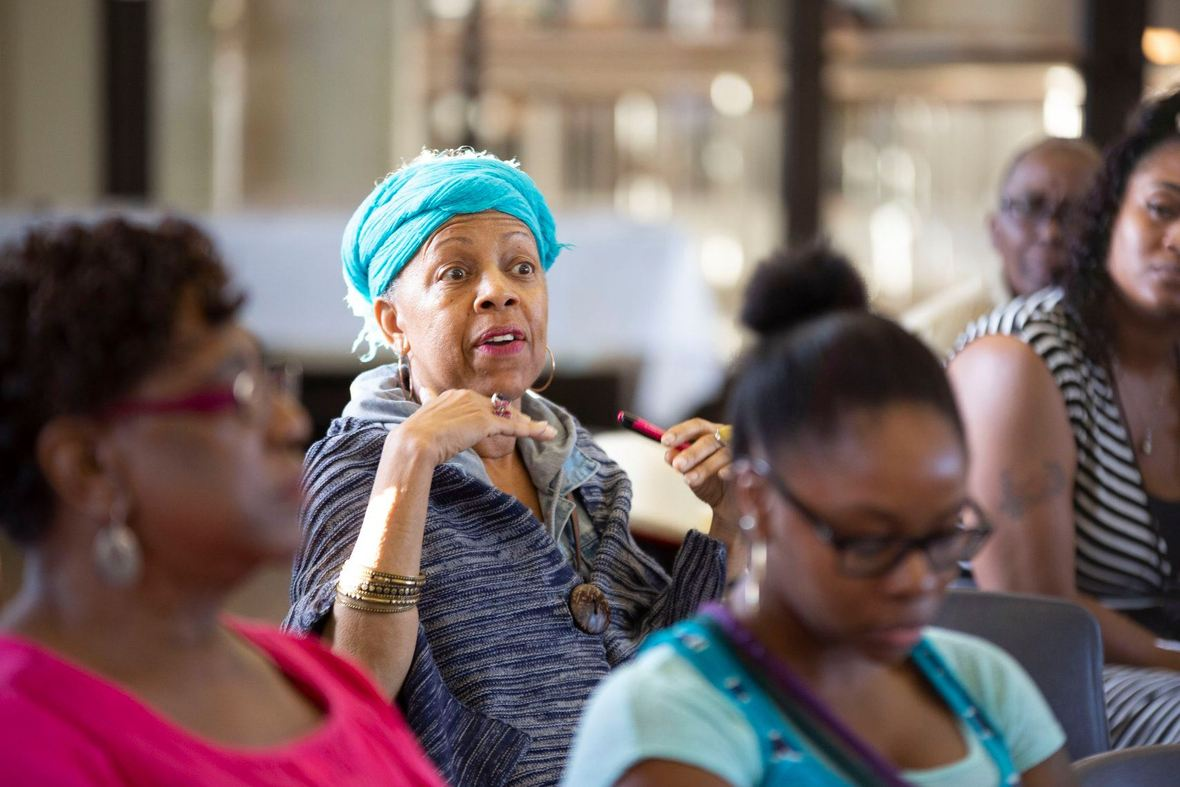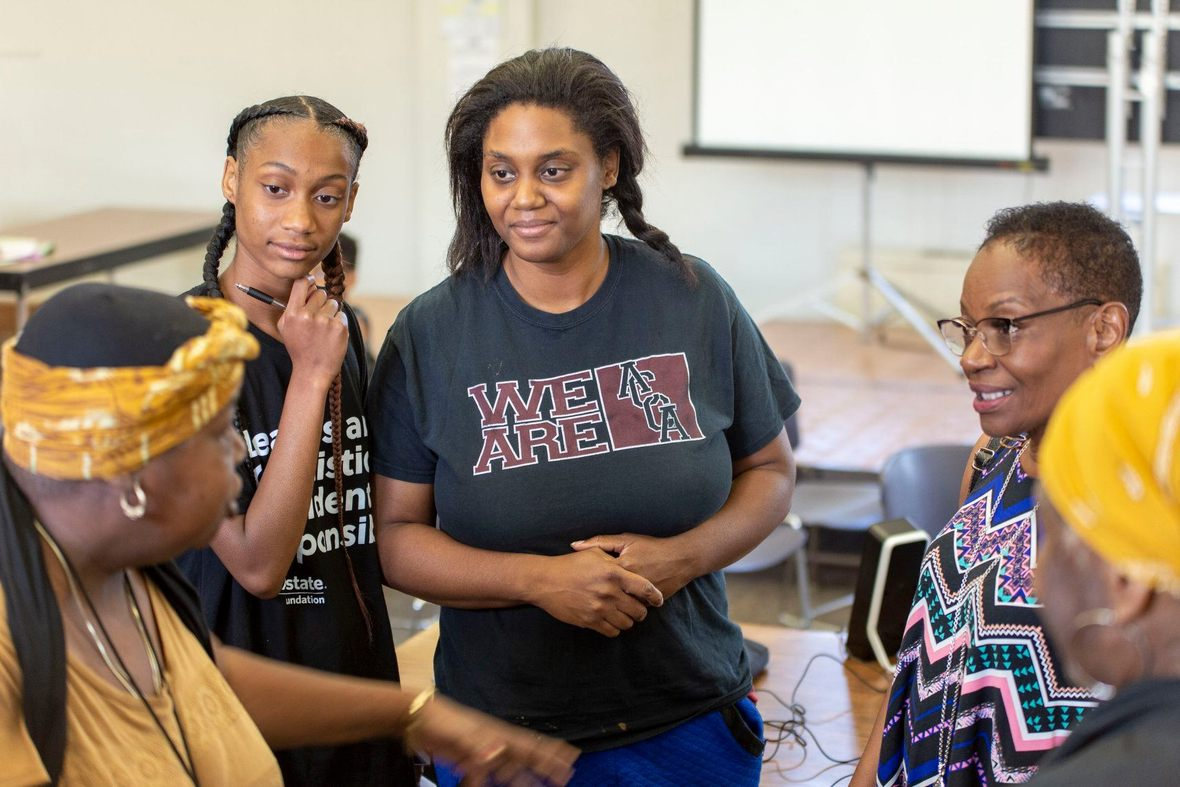/* styles */ The summer has kept conversations surrounding THE AREA going strong. We had another incredible screening at the Legler Regional Library in West Garfield Park this week on 7/15, as part of The Best of Black Harvest in Chicago Public Libraries series. Deborah and Brian participated in a lively post-screening discussion moderated by Keisha Chavers of Black Harvest Film Festival, exploring activism and empowerment in our communities with a West Side audience. We'd like to thank The Gene Siskel Film Center, the Legler Library and all those who attended for providing a space for screening and audience engagement.
 table div table+table+table+table+table+table+table+table+table+table div table{width:100%;padding:0}table div table+table+table+table+table+table+table+table+table+table div table img{width:96.23%;padding:0;float:none}table div table+table+table+table+table+table+table+table+table+table div table td{width:100%;padding:0 1.88% 18px}/* styles */## TUESDAY Bronzeville Historical Society Screening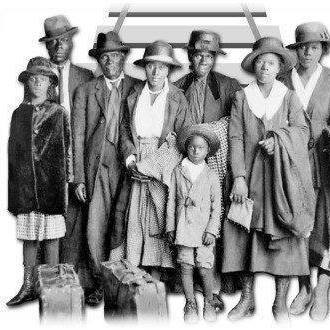/* styles */ THE AREA screens on 7/23 at the Mandrake Park Facility (3858 S. Cottage Grove Ave) hosted by The Bronzeville / Black Chicagoan Historical Society with support from the Burnham Park Advisory Council, Mandrake Park, The Partnership for Safe and Peaceful Communities Fund, and friends of Englewood, Bronzeville, and Woodlawn communities. Post-Screening Discussion Featuring: Sherry Williams, Bronzeville Historical Society President Deborah Payne, Protagonist & Co-Producer Brian Ashby, Co-Producer & Editor John Ellis, Englewood Activist Manny Frencha, Woodlawn Activist Yaaba X, Activist, Educator, Author __ This event is free and open to the public.
 table div table+table+table+table+table+table+table+table+table+table+table+table+table+table+table+table div table{width:100%;padding:0}table div table+table+table+table+table+table+table+table+table+table+table+table+table+table+table+table div table img{width:96.23%;padding:0;float:none}table div table+table+table+table+table+table+table+table+table+table+table+table+table+table+table+table div table td{width:100%;padding:0 1.88% 18px}/* styles */## Monday, 9/23 – The Area @ Comfort Station/* styles */ The Art Leaders of Color Network is hosting a free outdoor screening of THE AREA on 9/23 at Comfort Station (2579 N Milwaukee Ave, Chicago, IL 60647). ALCN's mission is to deliver critical insights and initiatives that enlighten and empower artists/ audiences/ critics/ producers/ venues/ funders to seek and support arts programming by creatives of color." More details coming soon via Comfort Station's website.
 table div table+table+table+table+table+table+table+table+table+table+table+table+table+table+table+table+table+table+table+table+table div table{width:100%;padding:0}table div table+table+table+table+table+table+table+table+table+table+table+table+table+table+table+table+table+table+table+table+table div table img{width:96.23%;padding:0;float:none}table div table+table+table+table+table+table+table+table+table+table+table+table+table+table+table+table+table+table+table+table+table div table td{width:100%;padding:0 1.88% 18px}/* styles */## Saturday 9/28 – The Area @ Beverly Arts Center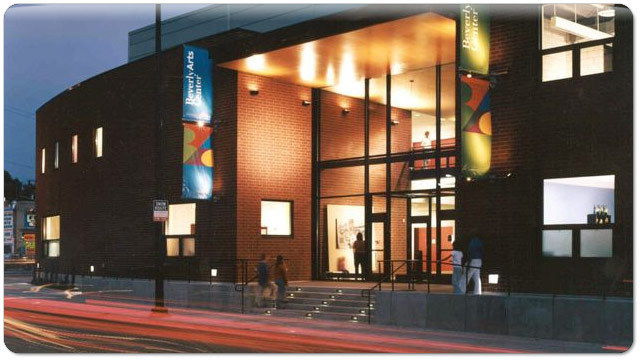/* styles */ Pullman Arts will screen THE AREA on 9/28 at The Beverly Arts Center (2407 West 111th Street Chicago, IL 60655) The Beverly Arts Center is, "a vibrant and multifaceted not-for-profit organization serving the Chicago metro area with high-quality programs in dance, visual arts, music, film and theater." Screening begins at 7:30pm. Tickets are \$6 and the screening will be followed by a Q&A with Deborah Payne (Protagonist and Co-Producer) and Brian Ashby (Co-Producer and Co-Editor) For more information, contact events@pullmanarts.org.
 table div table+table+table+table+table+table+table+table+table+table+table+table+table+table+table+table+table+table+table+table+table+table+table+table+table div table{width:100%;padding:0}table div table+table+table+table+table+table+table+table+table+table+table+table+table+table+table+table+table+table+table+table+table+table+table+table+table div table img{width:96.23%;padding:0;float:none}table div table+table+table+table+table+table+table+table+table+table+table+table+table+table+table+table+table+table+table+table+table+table+table+table+table div table td{width:100%;padding:0 1.88% 18px}/* styles *//* styles */ Nonprofit organization? Contact us for information on purchasing the institutional DVD of the film at a discount.
 table div table+table+table+table+table+table+table+table+table+table+table+table+table+table+table+table+table+table+table+table+table+table+table+table+table+table+table div table{width:100%;padding:0}table div table+table+table+table+table+table+table+table+table+table+table+table+table+table+table+table+table+table+table+table+table+table+table+table+table+table+table div table img{width:96.23%;padding:0;float:none}table div table+table+table+table+table+table+table+table+table+table+table+table+table+table+table+table+table+table+table+table+table+table+table+table+table+table+table div table td{width:100%;padding:0 1.88% 18px}/* styles */## Connect With Us

 table div table+table+table+table+table+table+table+table+table+table+table+table+table+table+table+table+table+table+table+table+table+table+table+table+table+table+table+table+table div table{width:100%;padding:0}table div table+table+table+table+table+table+table+table+table+table+table+table+table+table+table+table+table+table+table+table+table+table+table+table+table+table+table+table+table div table table{padding:0;float:left!important;width:35.714%!important}table div table+table+table+table+table+table+table+table+table+table+table+table+table+table+table+table+table+table+table+table+table+table+table+table+table+table+table+table+table div table td{padding-left:125px;padding-right:125px}table div table+table+table+table+table+table+table+table+table+table+table+table+table+table+table+table+table+table+table+table+table+table+table+table+table+table+table+table+table div table table+table{float:left!important;width:35.714%!important}table div table+table+table+table+table+table+table+table+table+table+table+table+table+table+table+table+table+table+table+table+table+table+table+table+table+table+table+table+table div table table td,table div table+table+table+table+table+table+table+table+table+table+table+table+table+table+table+table+table+table+table+table+table+table+table+table+table+table+table+table+table div table table+table td{padding-left:0;padding-right:20px}table div table+table+table+table+table+table+table+table+table+table+table+table+table+table+table+table+table+table+table+table+table+table+table+table+table+table+table+table+table div table table+table+table{float:left!important;width:28.572000000000003%!important}table div table+table+table+table+table+table+table+table+table+table+table+table+table+table+table+table+table+table+table+table+table+table+table+table+table+table+table+table+table div table table+table+table td{padding-left:0;padding-right:0}/* styles */
 table div table+table+table+table+table+table+table+table+table+table+table+table+table+table+table+table+table+table+table+table+table+table+table+table+table+table+table+table+table+table div table{width:100%;padding:0}table div table+table+table+table+table+table+table+table+table+table+table+table+table+table+table+table+table+table+table+table+table+table+table+table+table+table+table+table+table+table div table img{width:96.23%;padding:0;float:none}table div table+table+table+table+table+table+table+table+table+table+table+table+table+table+table+table+table+table+table+table+table+table+table+table+table+table+table+table+table+table div table td{width:100%;padding:0 1.88% 18px}/* styles *//* styles */ Kind regards, THE AREA Documentary Team
 table div table+table+table+table+table+table+table+table+table+table+table+table+table+table+table+table+table+table+table+table+table+table+table+table+table+table+table+table+table+table+table+table div table{width:100%;padding:0}table div table+table+table+table+table+table+table+table+table+table+table+table+table+table+table+table+table+table+table+table+table+table+table+table+table+table+table+table+table+table+table+table div table img{width:96.23%;padding:0;float:none}table div table+table+table+table+table+table+table+table+table+table+table+table+table+table+table+table+table+table+table+table+table+table+table+table+table+table+table+table+table+table+table+table div table td{width:100%;padding:0 1.88% 18px}/* styles */